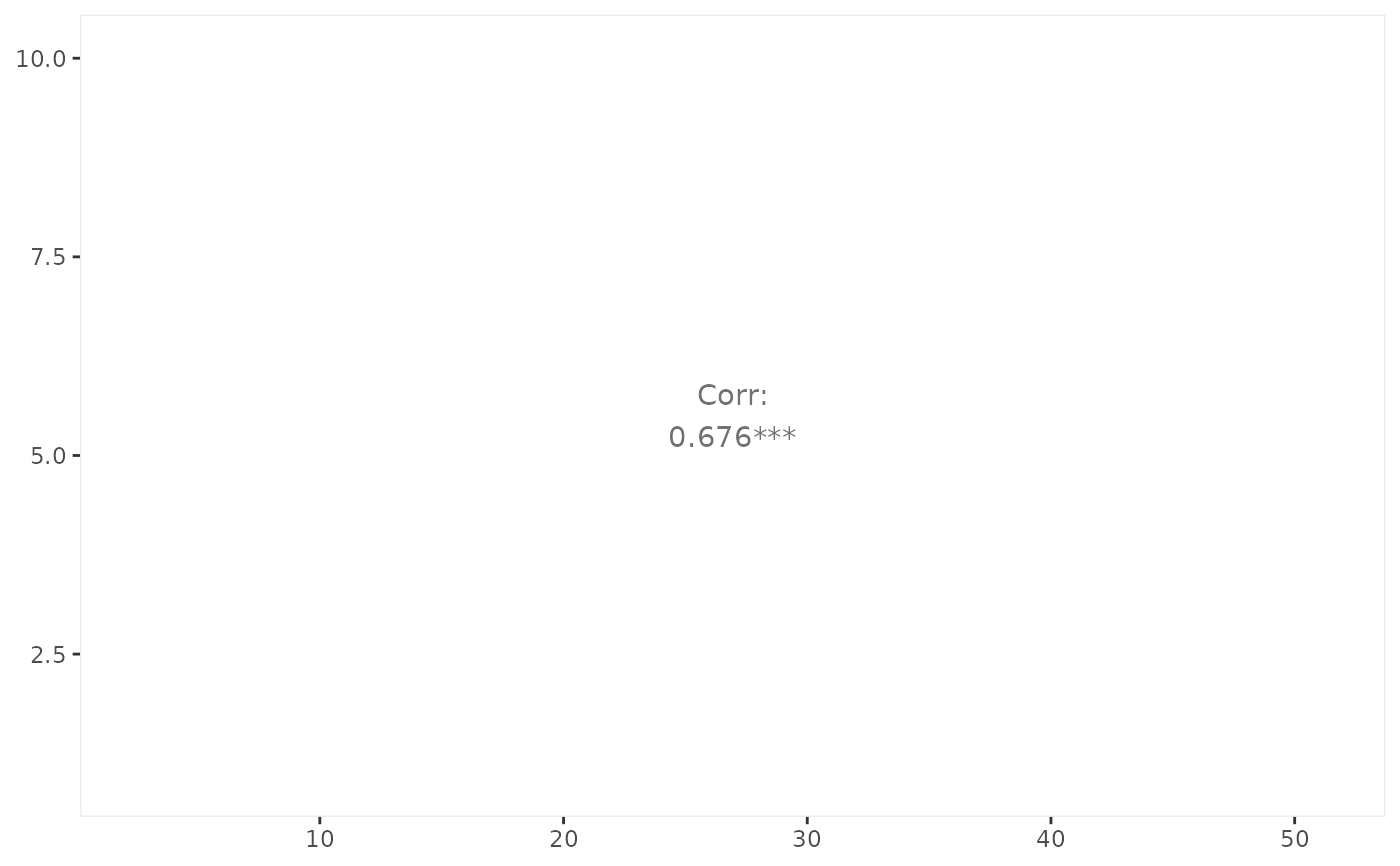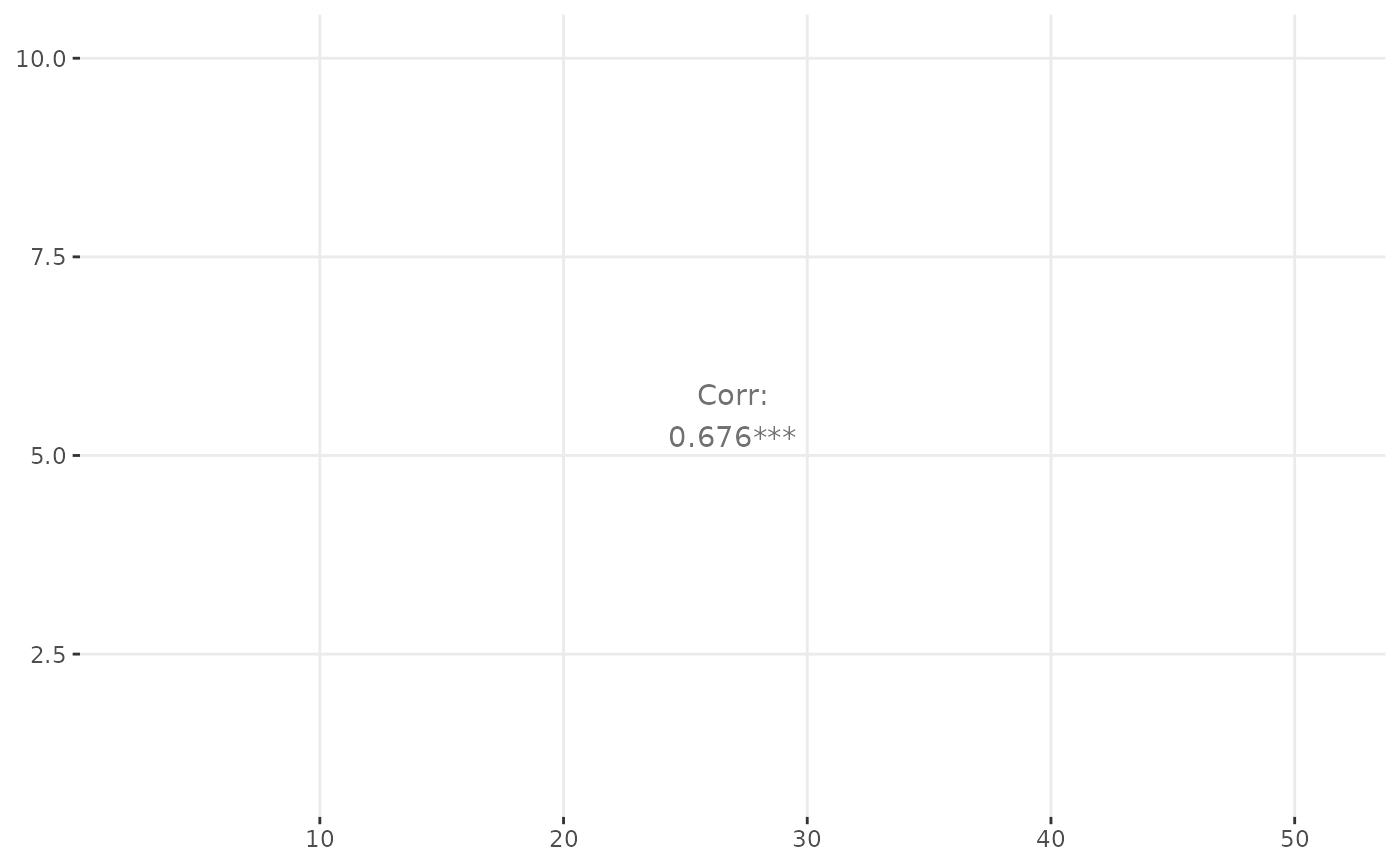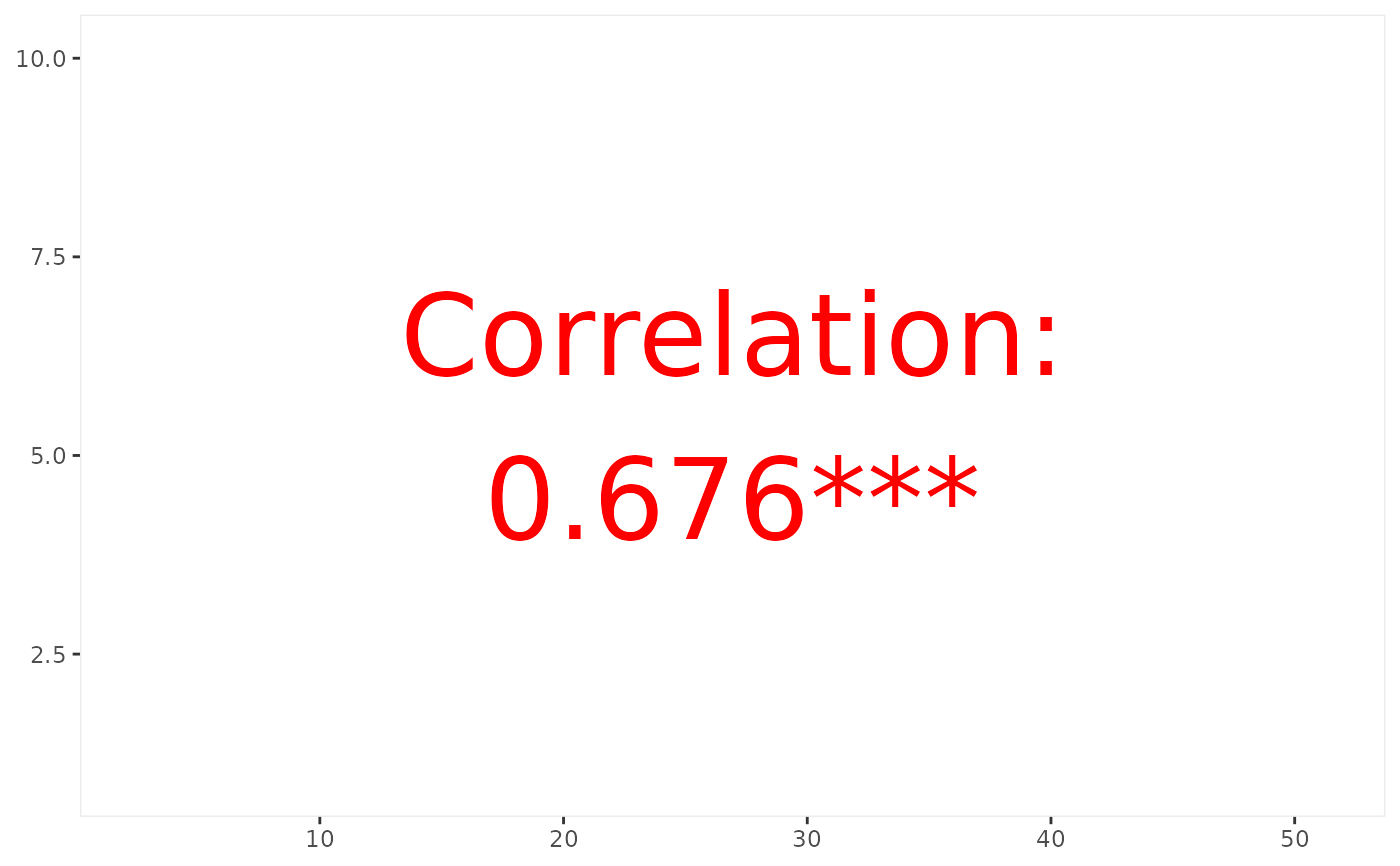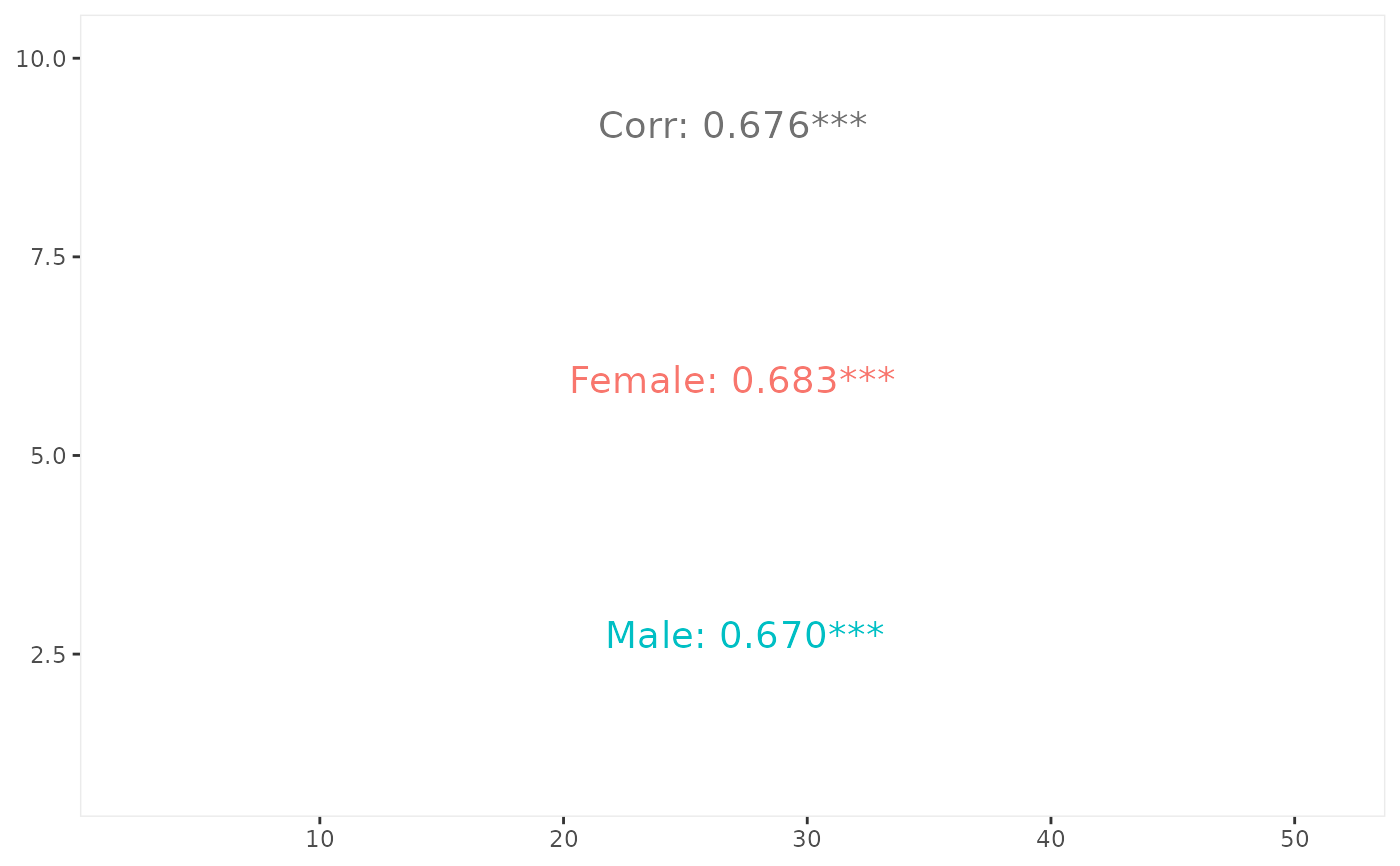Estimate correlation from the given data. If a color variable is supplied, the correlation will also be calculated per group.

ggally_cor(
data,
mapping,
...,
stars = TRUE,
method = "pearson",
use = "complete.obs",
display_grid = FALSE,
digits = 3,
title_args = list(...),
group_args = list(...),
justify_labels = "right",
align_percent = 0.5,
title = "Corr",
alignPercent = warning("deprecated. Use align_percent"),
displayGrid = warning("deprecated. Use display_grid")
)

## Arguments

data data set using aesthetics being used other arguments being supplied to geom_text() for the title and groups logical value which determines if the significance stars should be displayed. Given the cor.test p-values, display "***"if the p-value is < 0.001 "**"if the p-value is < 0.01 "*"if the p-value is < 0.05 "."if the p-value is < 0.10 ""otherwise method supplied to cor function use supplied to cor function if TRUE, display aligned panel grid lines. If FALSE (default), display a thin panel border. number of digits to be displayed after the decimal point. See formatC for how numbers are calculated. arguments being supplied to the title's geom_text() arguments being supplied to the split-by-color group's geom_text() justify argument supplied when formatting the labels relative align position of the text. When justify_labels = 0.5, this should not be needed to be set. title text to be displayed deprecated. Please use their snake-case counterparts.

ggally_statistic, ggally_cor_v1_5

Barret Schloerke

## Examples

# Small function to display plots only if it's interactive
p_ <- GGally::print_if_interactive

data(tips, package = "reshape")
p_(ggally_cor(tips, mapping = ggplot2::aes_string(x = "total_bill", y = "tip")))# display with grid
p_(ggally_cor(
tips,
mapping = ggplot2::aes_string(x = "total_bill", y = "tip"),
display_grid = TRUE
))# change text attributes
p_(ggally_cor(
tips,
mapping = ggplot2::aes(x = total_bill, y = tip),
size = 15,
colour = I("red"),
title = "Correlation"
))# split by a variable
p_(ggally_cor(
tips,
mapping = ggplot2::aes_string(x = "total_bill", y = "tip", color = "sex"),
size = 5
))